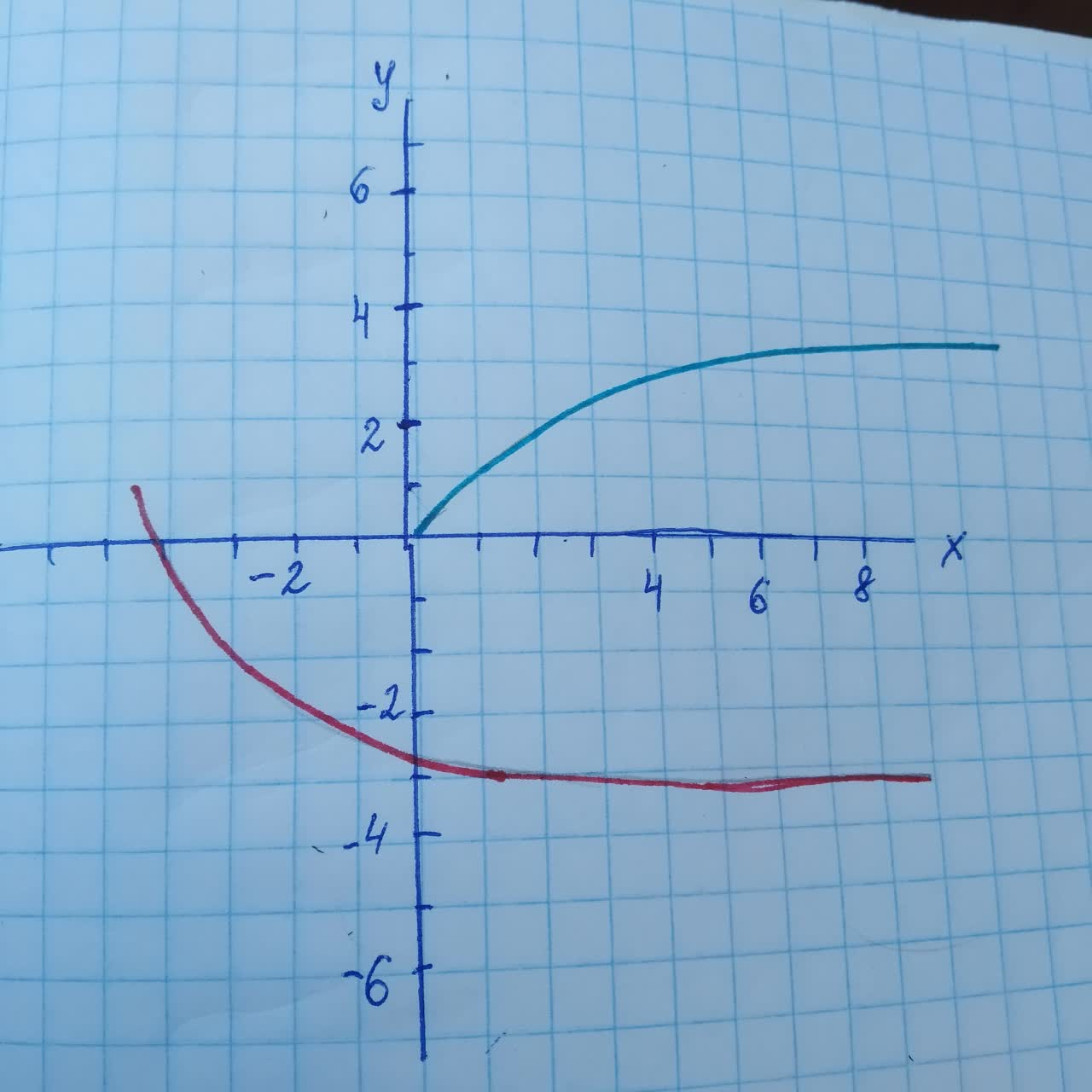Graph the function by hand, not by plotting points, but by starting with the graph of one of the standard functions, and then applying the appropriate transformations. y=1-2sqrt{x}+3permaneceerc 2021-01-07 Answered
Graph the function by hand, not by plotting points, but by starting with the graph of one of the standard functions, and then applying the appropriate transformations.
$$\displaystyle{y}={1}-{2}\sqrt{{{x}}}+{3}$$

• Questions are typically answered in as fast as 30 minutes

Solve your problem for the price of one coffee

• Math expert for every subject
• Pay only if we can solve itGennenzip
To plot the graph of the given function we will use the graph of $$\displaystyle{y}=\sqrt{{{x}}}.$$ We take the following steps. First we shift the graph of VT horizontally by 3 units to the left.This gives us the graph of m Next, we stretch the graph vertically by a factor of 2.After that, we reﬂect the graph around the x-axis.
Finally, we shift the graph of
$$\displaystyle-{2}\sqrt{{{x}+{3}}}$$
1 unit upward and obtain the graph of
$$\displaystyle{y}={1}-{2}\sqrt{{{x}+{3}}}$$
The hand-sketched graph is given bellow. Also we provide the graph obtained by using CAS.